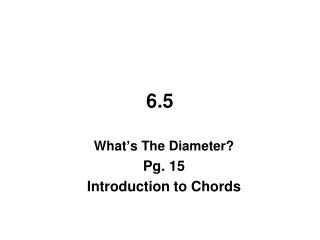DownloadDownload Presentation6.5

# 6.5

Download Presentation## 6.5

- - - - - - - - - - - - - - - - - - - - - - - - - - - E N D - - - - - - - - - - - - - - - - - - - - - - - - - - -
##### Presentation Transcript

1. 6.5 What’s The Diameter? Pg. 15 Introduction to Chords

2. What’s The Diameter? Introduction to Chords So far you learned that the diameter of a circle is the distance across the center of the circle. This length can be easily measured if the entire circle is in front of you and the center is marked, or if you know the radius of the circle. However, what if you only have part of a circle (called an arc)? Today you will consider a situation that demonstrates the need to learn more about the parts of a circle and the relationship between them.

3. 6.21 – THE WORLD'S WIDEST TREE The baobab tree (pronounced bay-O-bab) is a species of tree found in Africa and Australia. It is often referred to as the "world's widest tree" because it has been know to be up to 45 feet in diameter!

4. While digging at an archeological site, Rafi found a fragment of a fossilized baobab tree that appears to be wider than any tree on record! However, since he does not have the remains of the entire tree, he cannot simply measure across the tree to find its diameter. He needs your help to determine the radius of this ancient tree. Assume that the shape of the tree's cross-section is a circle.

5. The crease is the perpendicular bisector of

6. It is the diameter

7. 6.22 – GIVEN TWO CHORDS What if you know the lengths of two chords in a circle? How can you use the chords to find the center of the circle? Examine the chords provided below in the circles. Using only a compass and straightedge, find the center of each circle.

8. C

9. C

10. 5 8 5

11. 5 8 5

12. x2 + 42 = 52 4 5 x2 + 16 = 25 4 x2 = 9 5 x = 3